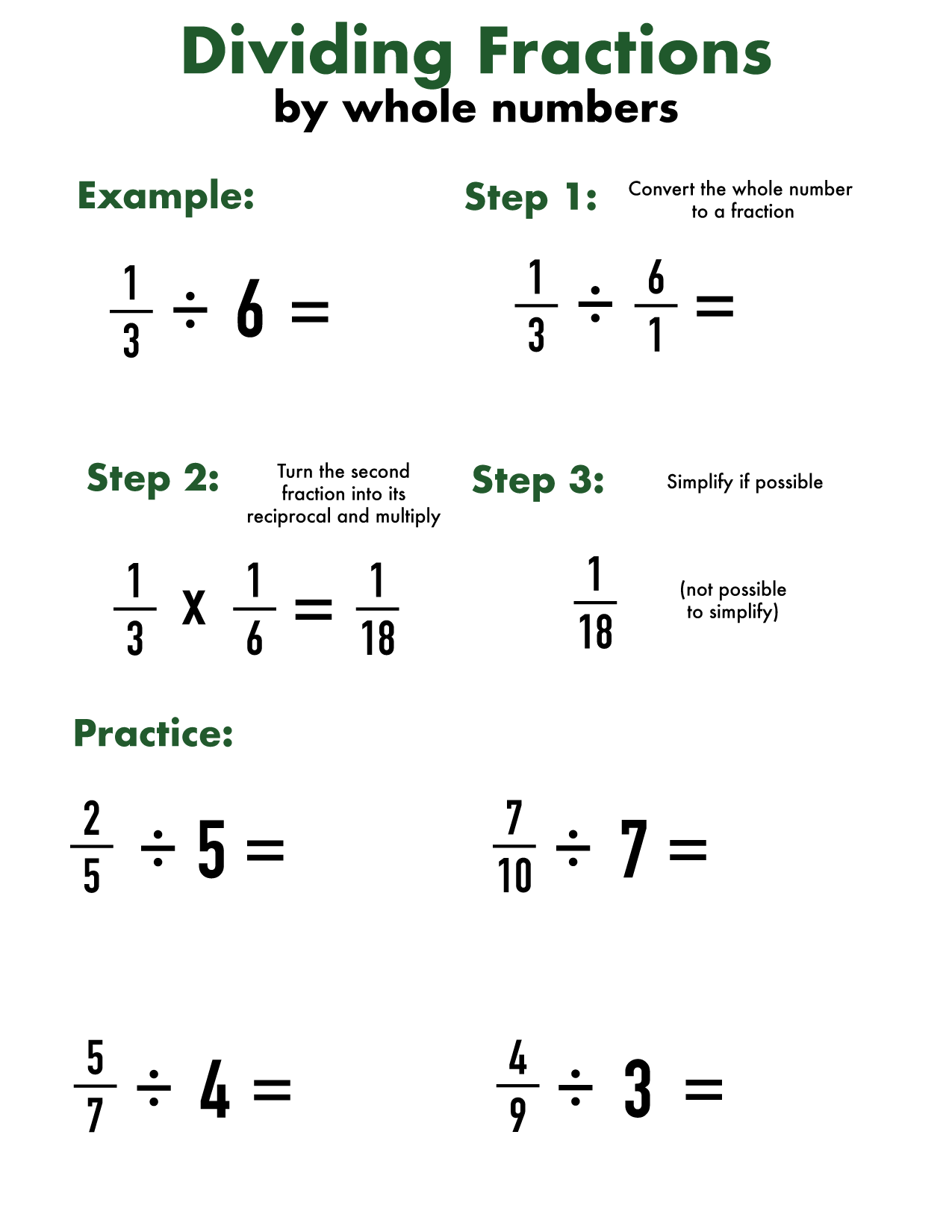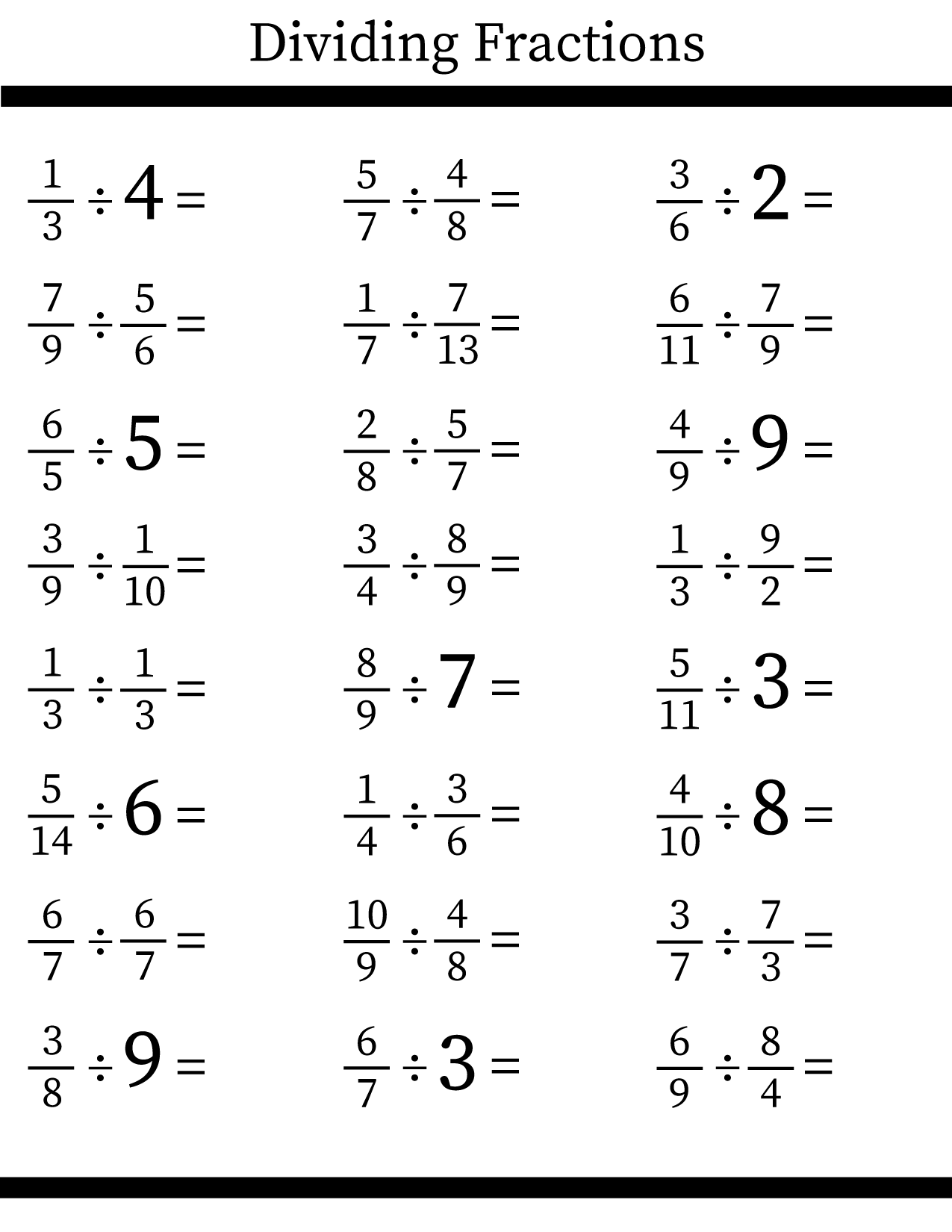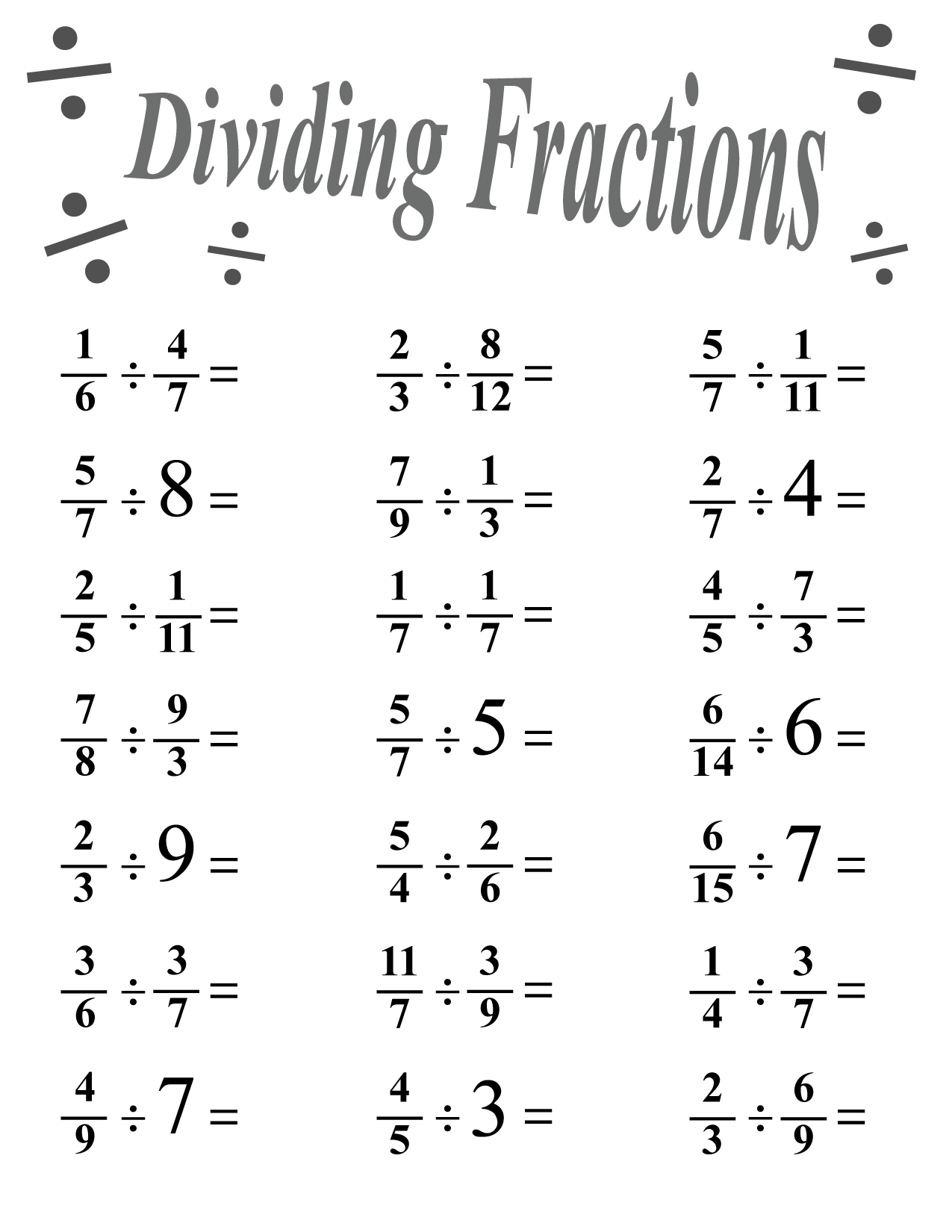Dividing fractions is the most difficult of functions involving fractions, but it’s not that hard once you get the hang of it! In this post, we will both give some explanation of dividing fractions, and then different worksheets for you to practice with!

If you need more worksheets after getting through these ones, have a look at this post on dividing fractions. If you want to practice multiplying fractions, we have worksheets on that here and here too.

## Dividing fractions by whole numbers #1## Dividing fractions by whole numbers #2

Our next worksheet is practice of what you’ve learned above. This sheet focuses on dividing by whole numbers, so students will have to make the whole numbers into fractions and then follow the rest of the steps.## Dividing fractions #1

This worksheet takes what the students have learned with whole numbers, and applies it to other fractions as well. The premise is the same; students will invert the second fraction to create its reciprocal, and then multiply. The final step is to simplify if possible.